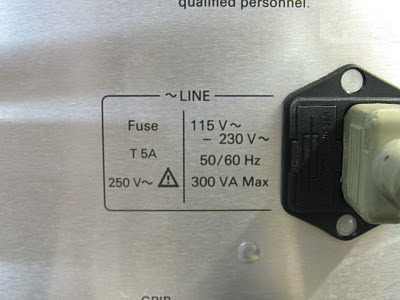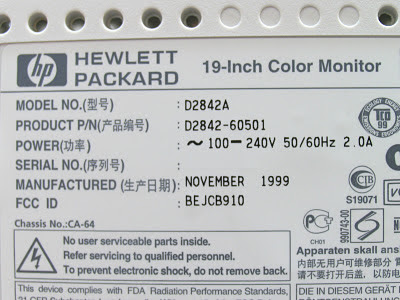## Monday, January 30, 2012

### Watts and volt-amperes ratings – what’s the difference and how do I choose an inverter based on them?

At the end of September, I posted about hurricane Irene and inverters. In that post (click here to read), I talked about the power ratings for inverters and just skimmed the surface about the differences between ratings in watts (W) and volt-amperes (VA). In this post, I want to go further into detail about these differences. Both watts and VA are units of measure for power (in this case, electrical). Watts refer to “real power” while VA refer to “apparent power”.

Inverters take DC power in (like from a car battery) and convert it to AC power out (like from your wall sockets) so you can power your electrical devices that run off of AC (like refrigerators, TVs, hair dryers, light bulbs, etc.) from a DC source during a blackout or when away from home (like when you are camping). Note that this power discussion is centered on AC electrical power and is a relatively short discussion about W, VA, and inverters. Look for a future post with more details about the differences between W and VA.

Watts: real power (W)
Watts do work (like run a motor) or generate heat or light. The watt ratings of inverters and of the electronic devices you want to power from your inverter will help you choose a properly sized inverter. Watt ratings are also useful for you to know if you have to get rid of the heat that is generated by your device that is consuming the watts or if you want to know how much you will pay your utility company to use your device when it is plugged in a wall socket since you pay for kilowatt-hours (power used for a period of time).

The circuitry inside all electronic devices (TVs, laptops, cell phones, light bulbs, etc.) consumes real power in watts and typically dissipates it as heat. To properly power these devices from an inverter, you must know the amount of power (number of watts, abbreviated W) each device will consume. Each device should show a power rating in W on it somewhere (390 W in the picture below) and you can just add the W ratings of each device together to get the total expected power that will be consumed. Most inverters are rated to provide a maximum amount of power also shown in watts (W) – they can provide any number of watts less than or equal to the rating. So, choose an inverter that has a W rating that is larger than the total number of watts expected to be consumed by all of your devices that will be powered by the inverter.Volt-Amperes: apparent power (VA)
VA ratings are useful to get the amount of current that your device will draw. Knowing the current helps you properly size wires and circuit breakers or fuses that supply electricity to your device. A VA rating can also be used to infer information about a W rating if the W rating is not shown on a device, which can help size an inverter. Volt-amperes (abbreviated VA) are calculated simply by multiplying the AC voltage by the AC current (technically, the rms voltage and rms current). Since VA = Vac x Aac, you can divide the VA rating by your AC voltage (usually a known, fixed number, like 120 Vac in the United States, or 230 Vac in Europe) to get the AC current the device will draw. To combine the apparent power (or current) of multiple devices, there is no straightforward way to get an exact total because the currents for each device are not necessarily in phase with each other, so they don’t add linearly. But if you do simply add the individual VA ratings (or currents) together, the total will be a conservative estimate to use since this VA (or current) total will be greater than or equal to the actual total.What if your device does not show a W rating?
Some electrical devices will show a VA rating and not a W rating. The number of watts (W) that a device will consume is always less than or equal to the number of volt-amperes (VA) it will consume. So if you need to size an inverter based on a VA rating when no W rating is shown, you will always be safe if you assume the W rating is equal to the VA rating. For example, assume 300 W for the 300 VA device shown in the picture above. This assumption may cause you to choose an oversized inverter, but it is better to have an inverter will too much capacity than one with too little capacity. An inverter with too little capacity will make it necessary for you to unplug some of your devices; otherwise, the inverter will simply turn itself off to protect its own circuitry each time you try to start it up, so it won’t work at all if you try to pull too many watts from it.

Some electrical devices will show a current rating (shown in amps, or A) and not a VA rating or W rating. Usually, this current rating is a maximum expected current. Maximum current usually occurs at the lowest input voltage, so calculate the VA by multiplying the current rating (A) times the lowest voltage shown on the device. Then, assume the device consumes an equal number of W as mentioned in the previous paragraph. For example, the picture below shows an input voltage range of 100 to 240 V and 2 A (all are AC). The VA would be the current, 2 A, times the lowest voltage, 100, which yields 200 VA. You could then assume this device consumes 200 W.1.Thank you for info.

2.Sorry, but I still do not understand the difference between VA and Watt. What is the difference between 'apparent' power and 'real' power? For me this should be the same for VxA=W... It looks like sales talk to me...? Like PMPO and RMS watts for audio... a sales gimmick.

1.Hello Anonymous,

I can assure you that the differences between W and VA are not at all controversial like the PMPO vs RMS issue as it applies to the audio industry. W and VA each have clear, indisputable mathematical definitions. Please refer to this article I wrote back in 2012 for those definitions and more information: http://electronicdesign.com/energy/what-s-difference-between-watts-and-volt-amperes

3.4.Hi,

Sorry to ask this question so much later after this post, if I had a product that was rated for 20W but it can go both on AC or DC power, how would I determine the VA exactly for the product when the datasheet only gives the watts and the current if I was to put the device on a transformer?

1.Hello Anonymous,

Using the device from a transformer implies an AC input. So if the datasheet uses this scenario to specify the AC current at a specific AC voltage, the VA rating is simply the product of these two numbers. The rating of 20 W alone does not give you any insight into the VA rating (other than it is >= 20) since you don't know the power factor (pf) which could be anything from 0 to 1. Since the definition of pf = W / VA, then VA = W / pf, so for your example, VA = 20 / some number between 0 and 1. As I mentioned above, this will yield any number >= 20.

I wonder why you want VA. Usually the VA number is used to calculate or estimate W and/or current, but you are already given those numbers, so you really don't need VA.

2.Hello Gary,

Its because I am trying to ensure I don't overload a transformer, but the transformer is rated for 70VA and I want to put the loads on my transformer, but all of the loads are rated in W